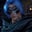Related Tags

# How to convert Int to Float and vice versa in HaskellGutha Vamsi Krishna

### Overview

We might need to convert from one data type to another data type while writing code. Most programming languages provide a way to convert data types explicitly using methods or functions.

### Convert Float to Int

In Haskell, we can convert Float to Int using the function round.

### Syntax

Let's view the syntax of the function.

round float_value
Round syntax

### Parameter

The round function takes the floating-point value as a parameter.

### Return value

It returns an integer value.

Let's look at an example of this.

### Code example

main::IO()

main = do
let num = 555.0009 :: Float
--convert float to int
print(round num)
Example for float to int conversion

### Code explanation

• Line 4: We declare and initialize the float variable num.
• Line 6: We get the integer value from the floating-point value using the round function, and display it.

### Convert Int to Float

In Haskell, we can convert Int to Float using the function fromIntegral.

### Syntax

Let's view the syntax of the function.

fromIntegral integer
The syntax for fromIntegral

### Parameter

The fromIntegral function takes an integer as a parameter.

### Return value

It returns a floating-point value.

Let's take a look at an example of this.

### Code example

main::IO()

main = do
let num = 99999 :: Int
--convert int to float
let new_num = fromIntegral num :: Float
print(new_num)

Example for int to float conversion

### Code explanation

• Line 4: We declare and initialize the integer num.
• Line 6: We convert int to float using the function fromIntegral and store the returned float value in the variable new_num.
• Line 7: We display the float value stored in the variable new_num.

RELATED TAGS

CONTRIBUTORGutha Vamsi Krishna
RELATED COURSES

View all Courses

Keep Exploring

Learn in-demand tech skills in half the time